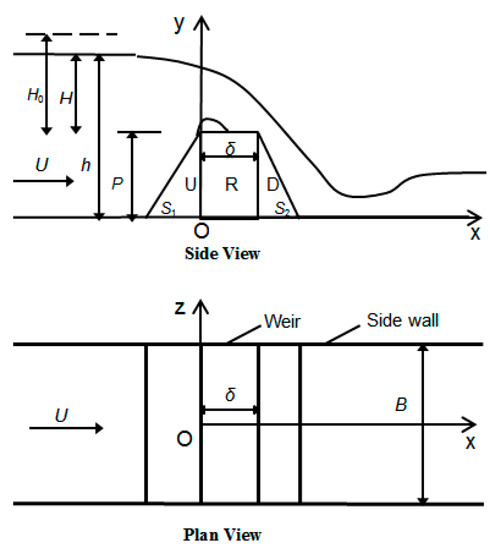Next Article in Journal
Prediction Method for the Complete Characteristic Curves of a Francis Pump-Turbine
Open AccessArticle

# Discharge Coefficient of Rectangular Short-Crested Weir with Varying Slope Coefficients

College of Water Conservancy and Hydropower Engineering, Hohai University, Nanjing 210098, China
*
Author to whom correspondence should be addressed.
Water 2018, 10(2), 204; https://doi.org/10.3390/w10020204
Received: 8 January 2018 / Revised: 7 February 2018 / Accepted: 11 February 2018 / Published: 14 February 2018
Rectangular short-crested weirs are widely used for simple structure and high discharge capacity. As one of the most important and influential factors of discharge capacity, side slope can improve the hydraulic characteristics of weirs at special conditions. In order to systemically study the effects of upstream and downstream slope coefficients S1 and S2 on overflow discharge coefficient in a rectangular short-crested weir the Volume of Fluid (VOF) method and the Renormalization Group (RNG) κ-ε turbulence model are used. In this study, the slope coefficient ranges from V to 3H:1V and each model corresponds to five total energy heads of H0 ranging from 8.0 to 24.0 cm. Comparisons of discharge coefficients and free surface profiles between simulated and laboratory results display a good agreement. The simulated results show that the difference of discharge coefficients will decrease with upstream slopes and increase with downstream slopes as H0 increases. For a given H0, the discharge coefficient has a convex parabolic relation with S1 and a piecewise linearity relation with S2. The maximum discharge coefficient is always obtained at S2 = 0.8. There exists a difference between upstream and downstream slope coefficients in the influence range of free surface curvatures. Furthermore, a proposed discharge coefficient equation by nonlinear regression is a function of upstream and downstream slope coefficients. View Full-Text
Show FiguresFigure 1

MDPI and ACS Style

Chen, Y.; Fu, Z.; Chen, Q.; Cui, Z. Discharge Coefficient of Rectangular Short-Crested Weir with Varying Slope Coefficients. Water 2018, 10, 204.

Show more citation formats Show less citations formats
Note that from the first issue of 2016, MDPI journals use article numbers instead of page numbers. See further details here.

1